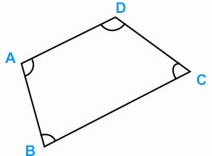HOME MATH DICTIONARY DOWNLOAD FEEDBACK DISCLAIMER
 Question: What is Opposite Angles ? Answer: When two straight lines intersect angles directly opposite each other, are equal and they are called opposite or vertical angles.In the quadrilateral ABCD, the angle at A and the angle at C are said to be opposite angles, as are the angles at B and D. In all parallelograms both pairs of opposite angles are equal. In a kite the two opposite angles on either side of the line of symmetry are equal.